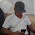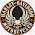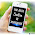4D Prediction Formula - GeoffreyStephen.com

# GeoffreyStephen.com

Search For Anything Tips Here (360°).

## Wednesday, April 15, 2015

Every day many people looking how to create a 4D prediction formula in lottery games in Malaysia and Singapore like Magnum 4D, Toto 4d Malaysia, Kuda 4d, 4D Singapore, Sabah 88 or lotto88, STC and Sarawak Cash Sweep.

In the Malay language known as "Nombor Ramalan 4D". I will teach you how to understand the mathematical formula to predict the 4D game. As a reminder, this formula is based solely on mathematical logic calculations and you should focus on making sure this counts really work in 4D games.

## The Best 4D Prediction Formula In Malaysia.

To understand the mathematical 4D formula, I will explain to you the basic math first. The basic numbers have ten different values of 0, 1, 2, 3, 4, 5, 6, 7, 8, 9. These numbers are divided into a number of odd and even numbers.

Even numbers are 0, 2, 4, 6, 8. Odd numbers are 1, 3, 5, 7, 9.

Well, do you understand to be here? Odd numbers and Even numbers must be separated into three figures.

Well, we will isolate the numbers "Even" first.

### Even= 24680

4680 = 680, 480, 460, 468
2680 = 680, 280, 260, 268
2480 = 480, 280, 240, 248
2460 = 460, 260, 240, 246
2468 = 468, 268, 248, 246

When separated this number, only 10 three-digit numbers are collected.

A) 680, 480, 460, 468, 280, 260, 268, 240, 248, 246

We have completed the separation of an even number, we separate the odd numbers.

### Odd= 13579

3579 = 579, 379, 359, 357
1579 = 579, 179, 159, 157
1379 = 379, 179, 139, 137
1359 = 359, 159, 139, 135
1357 = 357, 157, 137, 135

Three-digit number after the exile is

B) 579, 379, 359, 357, 179, 159, 157, 139, 137, 135Chart Formula
Try to see and observe the three-digit numbers with the chart above formula. For example, three Even numbers = 680. This combination of numbers is an Odd number = 1, 3, 5, 7, 9. It would be like this 6801, 6803, 6805, 6807, 6809. three odd numbers = 359. The combinations are even numbers = 2, 4, 6, 8, 0. it will be like 3592, 3594, 3596, 3598, 3590. This formula is actually the system 24, or numbers without coupling and this number is most often hit in the draw and still relevant.
If you see whenever a decision in Sports Toto Malaysia, Magnum 4D, DA, Ma CAI 1 + 3D, Sandakan Turf Club, Sabah88, Sarawak cash sweep and Singapore Pools 4D, three combinations of Odd and Even numbers will frequently hit the draw results 4D.

To understand this principle more clearly, numbers Even numbers are as follows, Even number is = AAAA. Odd numbers = BBBB. Formula is like this = AAAB, AABA, ABAA, BAAA, BBBA, BBAB, BABB, BBBB.

You just need to use the chart above 4D prediction formula to guide investment in the projected numbers. This formula can actually be used in all types of four-digit lotteries around the world because this formula is a mathematical fact.

Three even and odd numbers in the chart this formula has 20 numbers of 10 even and 10 odd. how to use this formula chart is buying 2 to 3 tickets, 4D lucky number and is priced at RM 1 only. If your ticket has a three-digit number as in the formula above, espouse with odd or even numbers.

Must Read: 4D Analysis Method

I hope you understand my explanation here. Use this formula only as a guide for me remind you any accuracy or reliability of this formula, I will not be responsible. A risk in your own hands. I just give you a little math fact and tips you need to know about this formula if you are an investor numbers 4d prediction. For better calculation using this formula, read the article, How to Calculate Your Percent 4D Prediction.

1.Good explanation. Can you please explain how did you get the Chart Table for M-K-T?!?

2.Great. Thanks for the guidance SIFU. Anyway, need to know also how the chart table is calculated.

3.Chart table has some complicated calculations and requires a clear explanation. This time, I can not explain how I made the calculation chart, but I have to explain how to use the chart in the Malay language blog. blog address, tips4d2u.blogspot.com. TQ

1.This comment has been removed by the author.

4.thanks a lot. This formula is very nice and open up my mind about the lottery 4d..tq bro..

5.hi..bro,that was a good explanation T.q
It would be much appreciated if you could provide the MKT chart a bit earlier time very helpful.

6.ok bro. I try my best to provide it..

7.I made this chart is based on research and analysis of past results..

8.hi , how u calculate your chart table ? i have no idea to get it .

9.hai..Do the calculations,.... the total oF number zero to nine during the latest draw, do a set of numbers, and create charts. The principle to create chart is simple, the number of lots to the total number slightly. (I will explain in detail later)

1.Mr geoffrey..
is it true the slightest number will be at the top and bottom chart? And top 3x3 number will be the biggest to the smallest.. Example
1
9 9 9
8 8 8
7 7 7
1

Is it right..mr geoffrey??

2.yes..its true :)

3.Tq bro..so much.will give it a test..

4.One more thing bro..if the lowest nombor is the same let say 8 and 1..what number should be top and bottom??

5.One more thing bro..if the lowest nombor is the same let say 8 and 1..what number should be top and bottom??

6.This comment has been removed by the author.

10.Got it bro...

0 = 9
1 = 8
2 = 10
3 = 7
4 = 8
5 = 13
6 = 8
7 = 8
8 = 9
9 = 12

The above set of numbers is actually from the last draw (Draw #: 472) where No. 3 appearance is less compared to other numbers so No. 3 will at top and bottom followed by the highest count which is 5, 9, and 2. Next will be 0, 8, 1 (where 0 = 9, 8 = 9 and 1 = 8 from the last draw). Correct me if I am wrong.

Thank you very much.

1.yes .. it is true..

2.Odd = 24680 & Even = 13579 is not true.
Odd = 13579 & Even = 24680 is true.
Why you wrote it wrongly????

3.sorry about that mistake .. I will fix it immediately .. thanks for your concern..

11.Anyone won any numbers using this technique ?

12.boleh tulis dalam bahasa malaysia tak

13.14.I have made an app to predict 4d